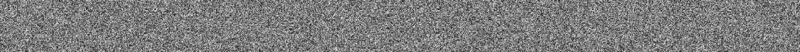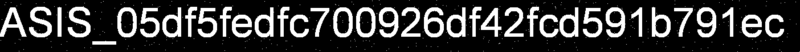ddaa's blog
Write-ups for CTF.
ddaa's blog

# ASIS CTF Crypto 150 Random Image

I didn't spend a lot of time at this CTF because I need to present my project about openstack. 217 is very powerful. When I joined the game, most of problems have been solved. I tried to solve the problem easy reading, but finally it was fruitless. (Nobody solved it.)

First, we downloaded the picture `enc.png` from problem. That is a black-and-white picture. Besite the picture, there is an encrypt program that is written by python.Observate the program, it loaded an image file into the object. Then the program created another imgae object that had the same size with the last one. After creating the object, the program filled the image with color from 0 to 249.

In following of codes, the program compared each pixel of original picture. If the color of pixel is smaller than 250, it will call `get_color()` to calculate other value and stored it into new image. Therefor, the other pixels are the pattern of the flag.

```def get_color(x, y, r):
n = (pow(x, 3) + pow(y, 3)) ^ r
return (n ^ ((n >> 8) << 8 ))
```

`r` is a random number from 1 to 2^256. However, after computing by `get_color`, it only had 256 values. We search a pixel with value bigger than 250, then try 256 times to break `r`.

```def get_r(e,x,y):
for r in range(256):
if get_color(x,y,r) == e:
return r
```

Once we get the value of `r`, we can seperate the flag and other pixels through expression `enpix[x,y] == get_color(x,y,r)`.